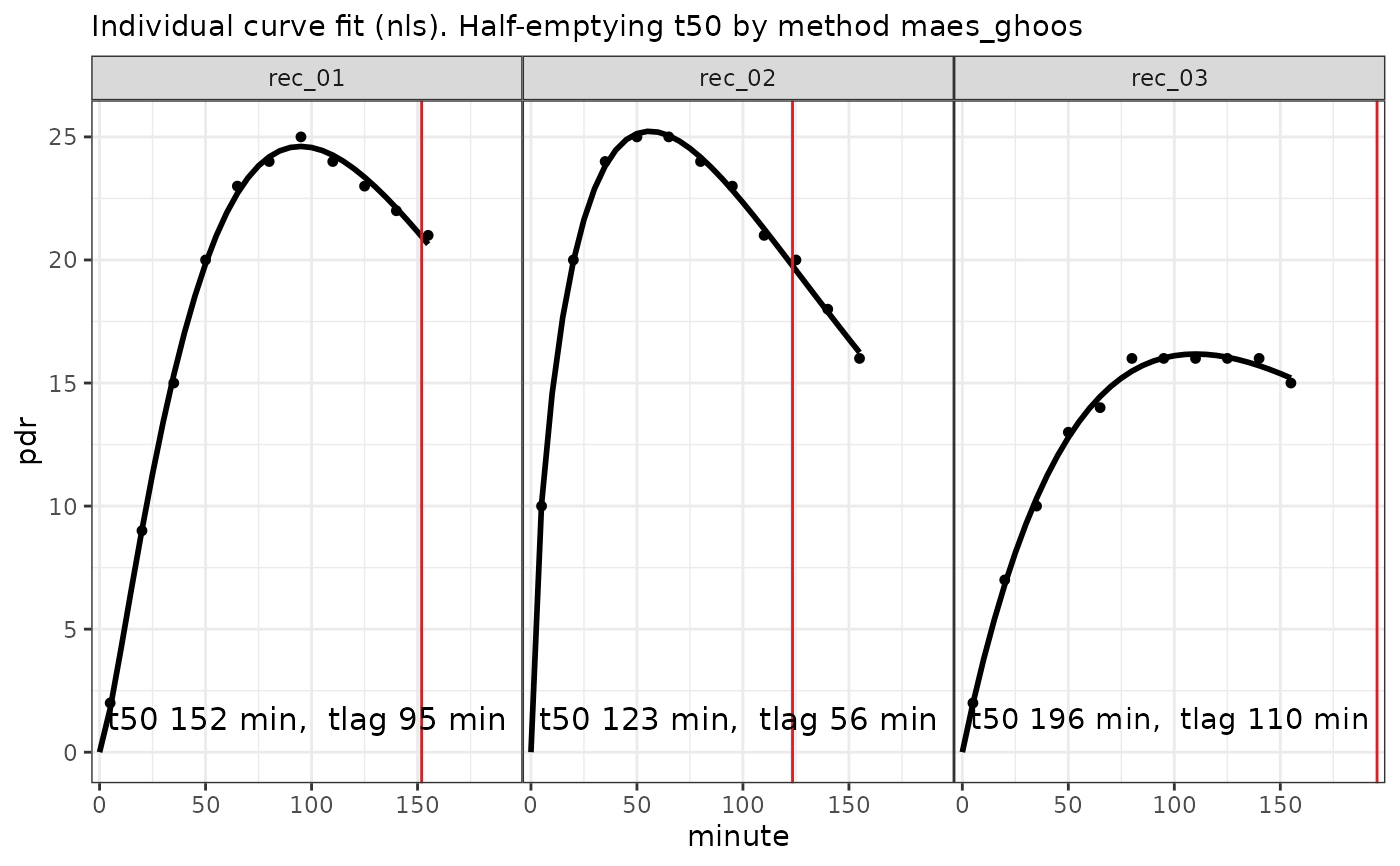Fits individual exponential beta curves to 13C breath test time series

## Usage

nls_fit(data, dose = 100, start = list(m = 50, k = 1/100, beta = 2))

## Arguments

data

Data frame or tibble as created by cleanup_data, with mandatory columns patient_id, group, minute and pdr. It is recommended to run all data through cleanup_data which will insert dummy columns for patient_id and minute if the data are distinct, and report an error if not.

dose

Dose of acetate or octanoate. Currently, only one common dose for all records is supported.

start

Optional start values patient_id and group.

## Value

A list of class ("breathtestnlsfit" "breathtestfit") with elements

coef

Estimated parameters in a key-value format with columns patient_id, group, parameter, stat, method and value. Parameter stat always has value "estimate". Confidence intervals might be added later, so do not take for granted all parameters are estimates.

data

Input data; nls_fit does not decimate the data. If you have large data sets where subsampling might be required to achieve faster convergence, using nls_fit anyway is only relevant to show how NOT to do it. Use nlme_fit or stan_fit instead.

Base methods coef, plot, print; methods from package broom: tidy, augment.

## Examples

d = simulate_breathtest_data(n_records = 3, noise = 0.2, seed = 4711)
data = cleanup_data(d$data) fit = nls_fit(data) plot(fit) # calls plot.breathtestfitoptions(digits = 2) cf = coef(fit) library(dplyr) cf %>% filter(grepl("m|k|beta", parameter )) %>% select(-method, -group) %>% tidyr::spread(parameter, value) %>% inner_join(d$record, by = "patient_id") %>%
select(patient_id, m_in = m.y, m_out = m.x,
beta_in = beta.y, beta_out = beta.x,
k_in = k.y, k_out = k.x)
#> # A tibble: 3 × 7
#>   patient_id  m_in m_out beta_in beta_out    k_in   k_out
#>   <chr>      <dbl> <dbl>   <dbl>    <dbl>   <dbl>   <dbl>
#> 1 rec_01        58  58.0    2.33     2.34 0.00898 0.00895
#> 2 rec_02        54  53.6    1.61     1.61 0.00847 0.00852
#> 3 rec_03        52  51.6    1.98     1.99 0.00623 0.00626